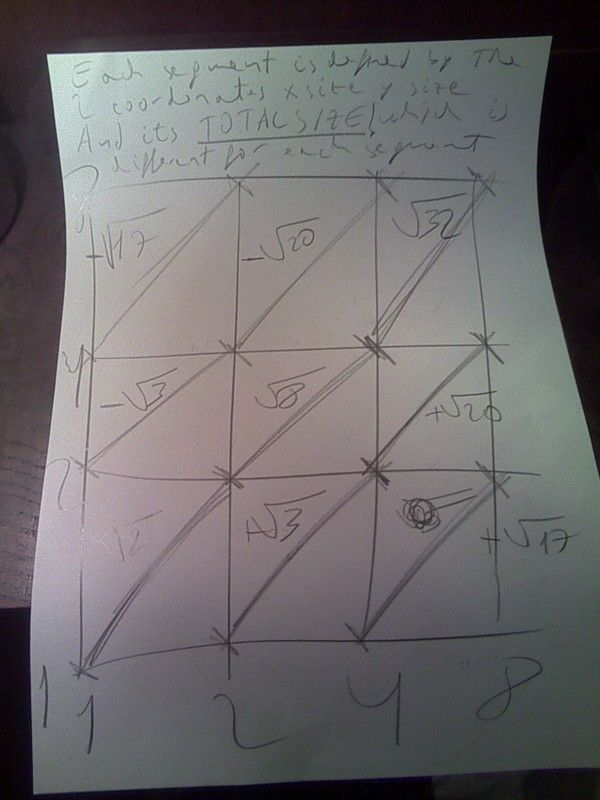#program to go from an euclidean space to a five dimentional one but i need to be able to make big powers• ### General discussion

•so basically clasically weve been considering motion is real and the change of size of objects as they aproach its an illusion

but i take it as motion its the illusion and change of size is real with which the 5 dimensions now are:

x rotation y rotation z rotation size and time

so i define an object of this space the apparent x size the apparent y size the apparent z size and the size we take as real

on this way this is what would happen with a segment in a plane and how instead of using 4 coordenates you just use 3:on this way i did this program which converts a fourdimensional space to five dimentional and back:

WGV699

but the problem i found is that small basic is very limited when calculating big powers

so maybe someone did a program to calculate powers with big numbers and addition with big numbers that would be so kind to let me use it?

im considering going to ruby for this but id rather do this in small basic

Saturday, December 31, 2016 2:07 AM

### All replies

•Hi Antonio !

if i take 5.1 multiply by ( 10 exponent 28 ) it work, Small Basic return OK

at 10 exponent 29 Small basic return a kind of overflow error., there is the limit.

like this:

___________________________________________________________________________

For t= 1 To 30
x=math.Power(10,t)*5.1

TextWindow.WriteLine (t)
TextWindow.WriteLine (x)

EndFor

__________________________________________________________________________

281474976710655 is the largest number Small Basic can handle

read in this forum : Small basic cannot handle large numbers?(simple question

• Edited by Saturday, December 31, 2016 2:32 AM
Saturday, December 31, 2016 2:25 AM
•Internally Small Basic uses the .Net type Decimal for numbers.  Check out its advantages and disadvantages including min max values.

https://msdn.microsoft.com/en-us/library/364x0z75(v=vs.140).aspx

https://www.dotnetperls.com/decimal

Saturday, December 31, 2016 11:22 AM